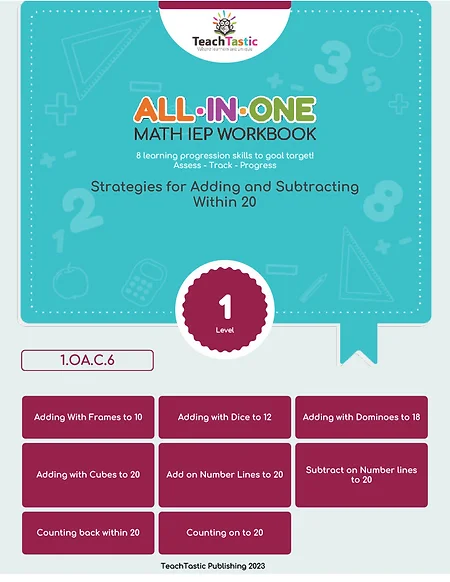top of page

# Lesson Plan

### First (1) - Math### LEARNING TARGET

• Students will be able to understand the commutative property of addition, that the order of the addends does not change the sum.
• Students will be able to use ten frames to solve addition problems to 10.
• Students will be able to write number sentences to represent addition problems.### LEARNING PROGRESSION

-

-

###### DURATION
• Introduction (5 minutes)
• Instruction (15 minutes)
• Guided Practice (15 minutes)
• Misconception Review (5 minutes)
• Independent Practice (15 minutes)
• Exit Card Formative Assessment (5 minutes)
###### MATERIALS
• Ten frames (at least 10)
• Connecting cubes (at least 50)
• Whiteboard and markers
###### VOCABULARY
• Commutative property
• Sum
• Ten frames### TEACHING RESOURCESNo Centers or Task Cards Available

###### IEP GOAL WORKBOOKSIntroducing the Strategies for Adding and Subtracting Within 20 workbook, designed for special education teachers who want innovative and practical ways to teach and scaffold learning for students with IEP goals. With a focus on individualized support, this workbook provides a clear and concise approach to mastering addition and subtraction within 20.

###### WORKSHEET PACK###### 5 AND 1 INTERVENTIONSNo Interventions Available

###### GAMES###### ACTIVITIESNo Activities Available### LESSON INSTRUCTION

###### INTRODUCTION
1. Review with students the concept of addition and how to use ten frames to solve addition problems to 10.
2. Introduce the commutative property of addition by explaining that it means you can change the order of the addends without changing the sum.
3. Give an example of the commutative property of addition, such as 3 + 2 = 2 + 3.
###### INSTRUCTION
1. Demonstrate how to use ten frames and connecting cubes to solve addition problems to 10, and how to write number sentences to represent the problems.
2. Model an example problem, such as 4 + 2 = ?, using the ten frames and connecting cubes.
3. Write the number sentence for the problem on the whiteboard: "4 + 2 = 6."
4. Emphasize the commutative property of addition by switching the order of the addends and showing that the sum is the same: "2 + 4 = 6."
###### GUIDED PRACTICE
1. Provide students with addition problem cards and have them use ten frames and connecting cubes to solve the problems.
2. Write the number sentences for each problem on the whiteboard as students complete them.
3. Encourage students to switch the order of the addends and show that the sum is the same.

###### INDEPENDENT PRACTICE
1. Give students a worksheet containing addition problems to 10.
2. Instruct students to use ten frames and connecting cubes to solve the problems and write number sentences to represent them.
3. Circulate around the room to provide support and guidance as needed.

###### HOMEWORK
1. Provide a worksheet for homework that reinforces the concept of the commutative property of addition. This can be a mix of addition problems with and without ten frames.
2. Encourage students to use the counters to help them solve the problems, and to check their work using the commutative property of addition.

###### EXIT TICKET
1. Provide each student with a whiteboard and marker.
2. Instruct students to write an addition problem and switch the order of the addends to show the commutative property of addition.
3. Observe students' responses to assess their understanding of the commutative property of addition and their ability to write number sentences to represent addition problems.

###### ASSESSMENT
1. Ask students to share an addition problem they solved and how they solved it, emphasizing the commutative property of addition.
2. Use their responses to assess their understanding of the commutative property of addition and their ability to write number sentences to represent addition problems.

###### CLOSURE
1. Review with students what they learned about the commutative property of addition and how to use ten frames to solve addition problems to 10.
2. Encourage students to practice using the commutative property of addition and ten frames to solve addition problems on their own.

###### EXTENSION
1. Students can practice solving addition problems with larger numbers on a ten frame.
2. Students can create their own addition problems and solve them using the ten frame and counters or the commutative property of addition.
###### INTERVENTION
1. For students who struggle with fine motor skills, larger and easier to grasp counters can be used.
2. For students who need extra support, a visual aid or diagram can be provided to help them understand the concept of the commutative properties of addition. Additionally, they can work in a small group with a teacher or teaching assistant for more individualized support.
###### VIDEOS

No Video Available### TEACHING TIPS

1. Use concrete examples and manipulatives to help students understand the commutative properties of addition.
2. Provide opportunities for students to practice using the ten frame and counters to solve addition problems.
3. Encourage students to explain their thought processes when solving problems, to promote deeper understanding.### STUDENT MISCONCEPTIONS

1. Addition is always done in a certain order, rather than recognizing that addition can be done in any order.
2. Using a ten frame is only helpful for small numbers, rather than recognizing that it can be used to represent larger numbers as well.
3. Not realizing that the commutative property of addition can be applied to all addition problems, not just specific ones.### STANDARD

##### Common Core Standard:

1.OA.C.6 - Add and subtract within 20, demonstrating fluency for addition and subtraction within 10. Use strategies such as counting on; making ten; decomposing a number leading to a ten; using the relationship between addition and subtraction.

bottom of page